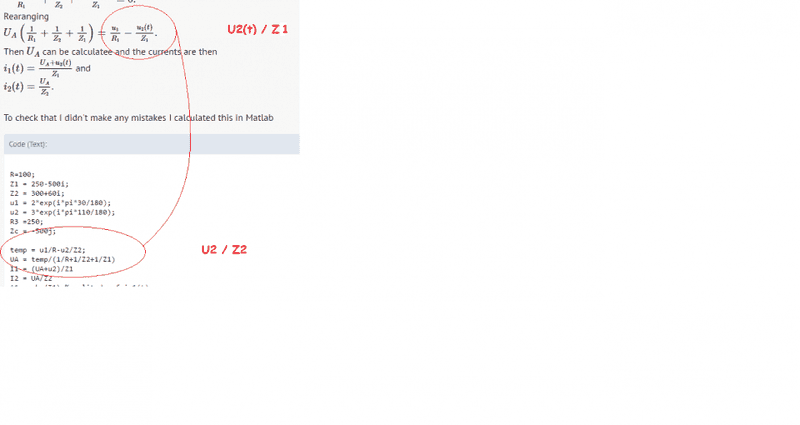# AC circuit analysis

## Homework Statement

Find the currents through ##R_3, i_1(t)## and ##R_2, i_2(t)##.
I attached a diagram of the circuit (diagram.pdf).

In the original diagram the currents I'm trying to find is marked going top to bottom, there's also no directions on the voltages in the original diagram but from the currents I guess they're in the direction drawn

##u_1(t) = 2\sin(200t+30^\circ)##
##u_2(t) = 3 \cos(200t + 20^\circ)##
##R_1 = 100 \Omega##
##R_2=300 \Omega##
##R_3 = 250 \Omega##
##C=10 \mu F##
##L=300mH##

N/A

## The Attempt at a Solution

Lets first simplify at bit by calculating the impedance.
We then have
##Z_1 = R_3+\frac{1}{j\omega C} = 250-j500##
##Z_2 = R_2+j\omega L = 300+j60##

We then have the diagram (diagram2.pdf)
We also write the voltages in complex form
##u_1(t) = 2e^{200t+30^\circ}##
##u_2(t) = 3e^{200t+110^\circ}.## (add ##90^\circ## to write the ##\cos## in ##\sin## form.)

Node analysis:
I ground the bottom node then I use KCL on the top Node, let's call it ##A## and it's potential be ##U_A##.
We then get the equation
##\frac{U_A-u_1(t)}{R_1} + \frac{U_A}{Z_2}+\frac{U_A+u_2(t)}{Z_1}=0.##
Rearanging
##U_A\left( \frac{1}{R_1} +\frac{1}{Z_2} + \frac{1}{Z_1} \right) = \frac{u_1}{R_1}-\frac{u_2(t)}{Z_1}.##
Then ##U_A## can be calculatee and the currents are then
##i_1(t) = \frac{U_A+u_2(t)}{Z_1}## and
##i_2(t) = \frac{U_A}{Z_2}##.

To check that I didn't make any mistakes I calculated this in Matlab
Code:
R=100;
Z1 = 250-500i;
Z2 = 300+60i;
u1 = 2*exp(i*pi*30/180);
u2 = 3*exp(i*pi*110/180);
R3 =250;
Zc = -500j;

temp = u1/R-u2/Z2;
UA = temp/(1/R+1/Z2+1/Z1)
I1 = (UA+u2)/Z1
I2 = UA/Z2
A1 = abs(I1) %amplitude of i_1(t)
phi1 = 180/pi*atan(imag(I1)/real(I1)) %phase constant of i_1
A2 = abs(I2)
phi2 = 180/pi*atan(imag(I2)/real(I2))

Using this I get the output
##UA = 1.3377 - 0.0697i##
##I1=-0.0041 + 0.0027i##
##I2 = 0.0042 - 0.0011i##
##A1 = 0.0049##
##A2 = 0.0044##
##phi1 = -33##
##phi2 = -14.3##
So the currents should be
##i_1(t) =4.9 \sin (200 t -33^\circ) mA## and
##i_2(t) = 4.4 \sin (200t - 14.3^\circ mA##.

However the answer says that it should be
##i_1(t) = 5.13\sin(200t + 15.7^\circ )## mA and
##I_2(t) = 3.73 \sin (200t + 26.2^\circ )##mA.

I've been at this question for two days and I can't see where I'm going wrong.

#### Attachments

• diagram.pdf
26.1 KB · Views: 150
• diagram2.pdf
25.1 KB · Views: 158
Last edited:

Merlin3189
Homework Helper
Gold Member
Only thing I've noticed so far:•Incand
Nice catch! That together with changing the sign of one of the voltage sources fixes it. Apparently one of them was meant to be the other way around.